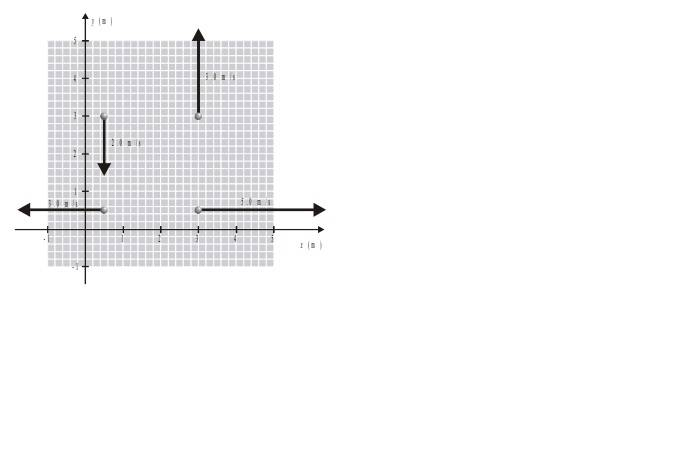# Calculate Internal Angular Momentum and Energy

Nicolas Gallardo

## Homework Statement

Four particles of mass 1 Kg each, are moving on a plane with the velocities given in the figure.## The Attempt at a Solution

First I calculated the position of the CoM:
Xcm=7/4(i + j)
Then I calculated the velocity of the CoM:
Vcm= ½i + ¼j

For the internal energy I did the following:

Kint= (1/2)(1.52) + (1/2)(1.32) + (1/2)(1.32) + (1/2)(1.22) = 23,5J

But the answer is 22,9 J. And for the internal angular momentum I do not actually know with respect to which point I should calculate it. Any hint would be appreciated. Thank you.

#### Attachments

Last edited:

Homework Helper
Gold Member
Xcm=7/4(i + j)
Then I calculated the velocity of the CoM:
Vcm= ½i + ¼j

For the internal energy I did the following:

Kint= (1/2)(1.52) + (1/2)(1.32) + (1/2)(1.32) + (1/2)(1.22) = 23,5J
The numbers in the figure are hard to read. If I'm reading them correctly, it appears that your results for the position and velocity of the center of mass are correct. But, it doesn't appear to me that your values for the "internal" speeds of the particles are correct. For example, how did you get 1.5 m/s for the speed that you used in the first term of Kint? To which particle does this refer?

Nicolas Gallardo
The numbers in the figure are hard to read. If I'm reading them correctly, it appears that your results for the position and velocity of the center of mass are correct. But, it doesn't appear to me that your values for the "internal" speeds of the particles are correct. For example, how did you get 1.5 m/s for the speed that you used in the first term of Kint? To which particle does this refer?

That is (1/2)(mv^2)⇒ (1/2)(1(5^2)). One of the velocities is 5 m/s.
The other 3 velocities are: 3 m/s, 3 m/s, 2m/s

Homework Helper
Gold Member
That is (1/2)(mv^2)⇒ (1/2)(1(5^2)). One of the velocities is 5 m/s.
5 m/s is the speed of the particle relative to the reference frame of the Cartesian axes shown in the picture. You would use 5 m/s if you wanted the kinetic energy of the particle relative to this frame. But, you are looking for the "internal kinetic energy". This would be the kinetic energy relative to what frame of reference?

Nicolas Gallardo
5 m/s is the speed of the particle relative to the reference frame of the Cartesian axes shown in the picture. You would use 5 m/s if you wanted the kinetic energy of the particle relative to this frame. But, you are looking for the "internal kinetic energy". This would be the kinetic energy relative to what frame of reference?
Relative to the CoM? But how would that change in the equation?

Homework Helper
Gold Member
Relative to the CoM?
Yes
But how would that change in the equation?
Can you find the velocity of each particle relative to the CoM frame?

Nicolas Gallardo
Yes
Can you find the velocity of each particle relative to the CoM frame?
If we denote V' to the velocity of the particle related to the CoM frame, V to the velocity of the particle related to the cartesian axe and VCoM to the velocity of the CoM, then the velocity of the particle would be:
V'= Vcartesian - VCoM
Am I correct?